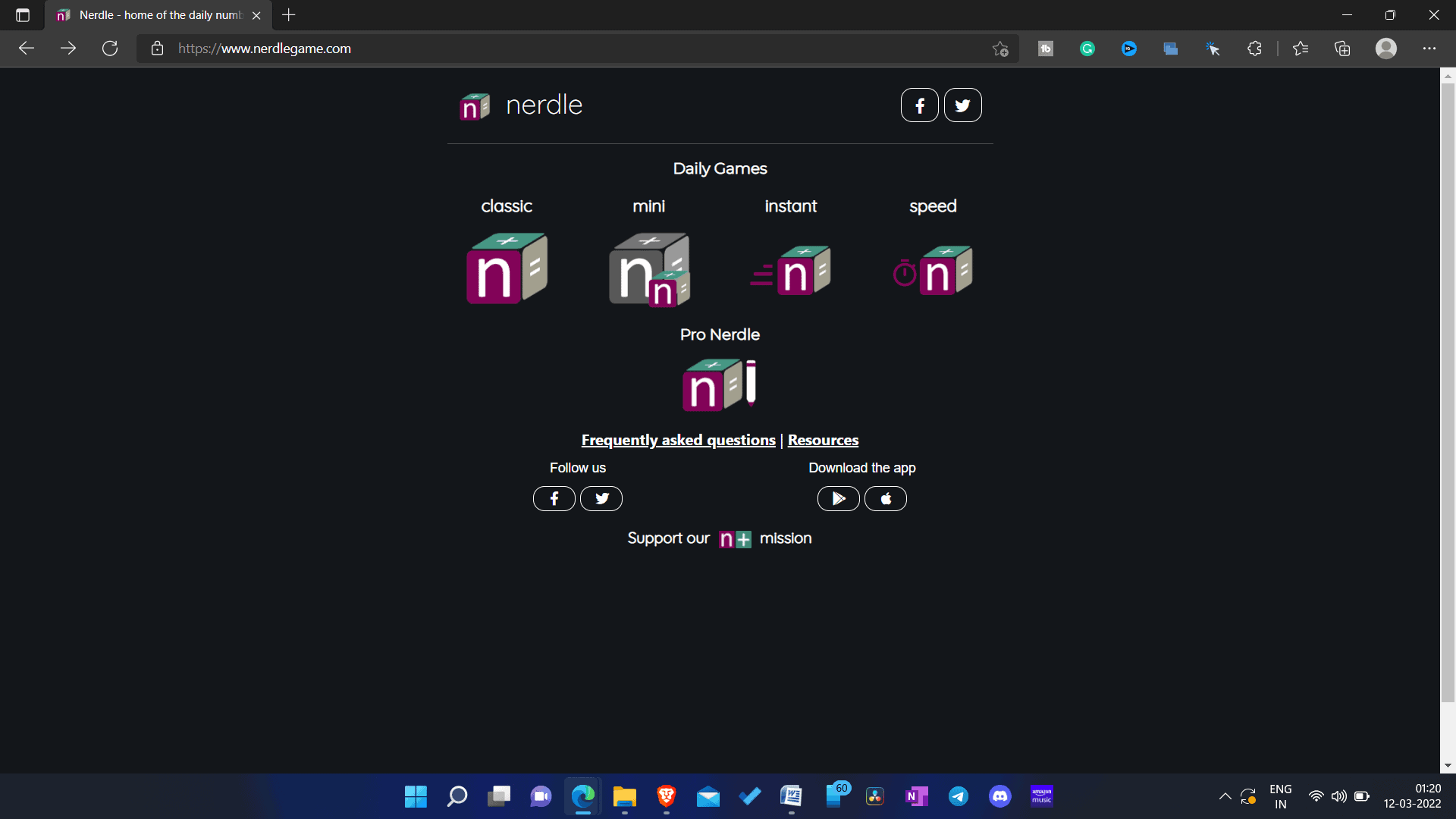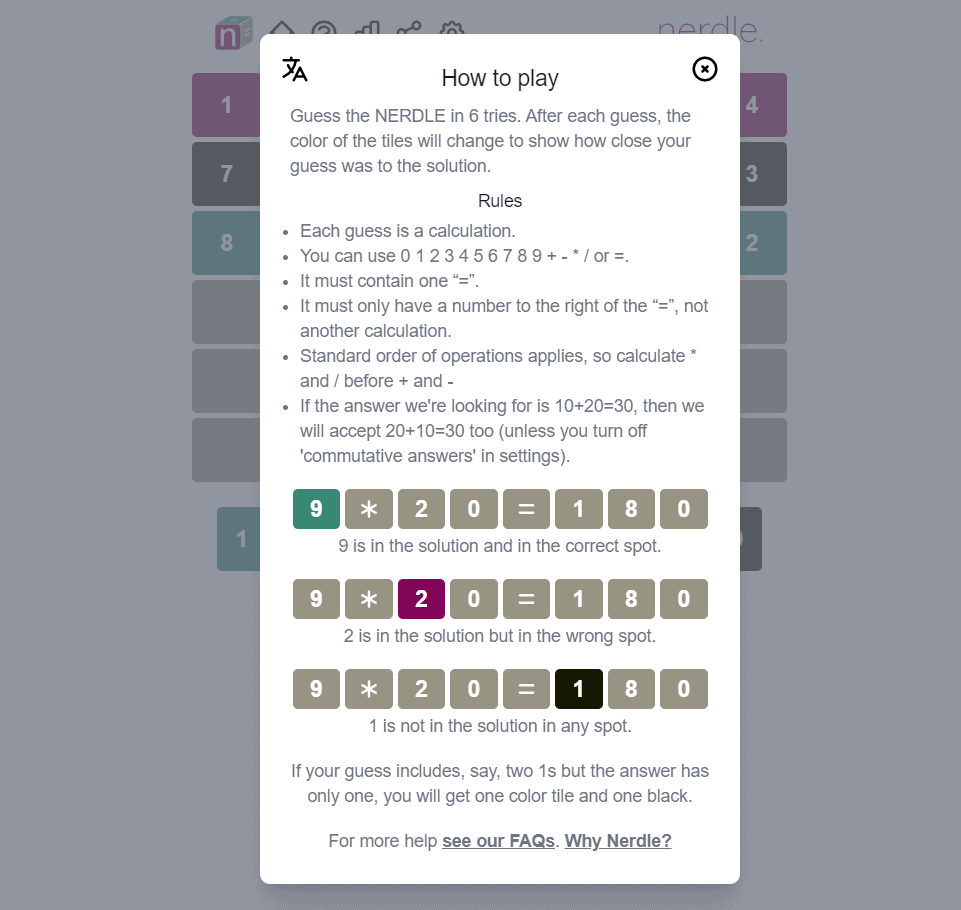Monday, December 4, 2023

-

Since Wordle became popular, people have come up with new alternatives customizations and features. One such alternative that developers have come up with is Nerdle. Nerdle is a number game where the player will guess the correct mathematic equation. The game has six tries in which you will have to guess the correct Wordle equation. It gets challenging to guess the correct equation within six attempts, and you may lose the game. If you want to keep up with your Nerdle streak, this article will help you.

Contents

## How To Play Nerdle Game?

If you are playing the game for the first time, and you don’t know how to do it, then you can follow the instructions given below-

• Click on this link to head to the Nerdle website. Next, you can select between the following modes- Mini Nerdle, Classic Nerdle, Instant Nerdle, Speed, and Pro Nerdle.• Depending on the mode you select, you will have to guess the correct Nerdle within six tries or two tries.
• The color of the tiles will change depending on the number you typed and how close it was to that day’s Wordle.

## Nerdle RulesThere are certain rules that you will have to keep in mind before playing the game. Ensure you follow the Nerdle rules given down below to play the game correctly-

• Each guess or try is the calculation.
• You can use numbers (0 1 2 3 4 5 6 7 8 9 0) or calculations symbols (+ – * / =)
• An equation must have single = and note more than that.
• There should be a number at the right of the = and not another calculation.
• Standard mathematic operation rule is followed, so you will have to multiply or divide before adding or subtracting.
• Commutative answers are also accepted unless you turn them off manually.

## Full Nerdle Answers 4th December 2023

Given below are all is the list of all the Nerdle answers for classic Nerdle as well as mini Nerdle-

• Nerdle Day 51 — March 12, 2022 — 8*4/16=2
• Nerdle Day 50 — March 11, 2022 — 73-10=63
 Date Mini Nerdle Classic Nerdle Instant Nerdle 10th March 2022 Day 50 6*6=36 73-10=63 37-8*4= 5 9th March 2022 Day 49 16-8=8 54+34=88 156/39=4 8th March 2022 Day 48 8+7=15 60/5-6=6 9-42/7= 3 7th March 2022 Day 47 49/7=7 648/8=81 5-18/6=2 6th March 2022 Day 46 8*6=48 5+12-9=8 9+7*6=51 5th March 2022 Day 45 14-5=9 84/6-6=8 3+16/4=7 4th March 2022 Day 44 5+6=11 42*7=294 3+18/9=5 3rd March 2022 Day 43 48/6= 8 11-1-3=7 5-14/7= 3 2nd March 2022 Day 42 20/4= 5 6*6-2= 34 3+21/7= 6 1st March 2022 Day 41 9*4= 36 58-41=17 52*7=364 28th Feb 2022 Day 40 4*6=24 6-8+10=8 2+15/3= 7 27th Feb 2022 Day 39 18/9=2 98-54=44 9-42/7= 3 26 Feb 2022 Day 38 8+5= 13 3*42= 126 63/9+1= 8 25th Feb 2022 Day 37 15/3=5 100-98=2 261/3= 87 24th Feb 2022 Day 36 8*4= 32 94-42=52 84/7-9= 3 23rd Feb 2022 Day 35 25/5= 5 6+6+7= 19 1+42/6=8 22nd Feb 2022 Day 34 5*9= 45 36+12= 48 5-24/6= 1 21st Feb 2022 Day 33 9*7=63 1-8+16=9 368/92=4 20th Feb 2022 Day 32 9 * 6 = 54 89 * 3 = 267 9 / 3 * 4 = 12 19th Feb 2022 Day 31 54 / 9 = 6 6*6+4 = 40 1+54/9 = 7 18th Feb 2022 Day 30 2 * 7 = 14 88 – 25 = 63 25 – 3 * 6 = 7 17th Feb 2022 Day 29 15 – 7 = 8 67 – 32 = 35 4 * 39 =156 16th Feb 2022 Day 28 30 / 5 = 6 20 – 6 – 5 = 9 5 – 32 / 8 = 1 15th Feb 2022 Day 27 3 * 8 = 2 4 7 + 7 / 1 = 1 4 5 7 * 6 = 3 4 2

## Final Words

These were all the Nerdle answers and even the answer for the current date. We have also discussed how you can play the game and the rules to help you play and win the game.

RELATED GUIDES:Anurag Jainhttps://itechhacks.com
Anurag is a Senior content analyst with 4 years of experience in the industry. Based in India, He is extremely skilled at Windows related to How to Troubleshooting matters. His favorite topics are Windows 11, Android, and How To's.